# Circles

Three circles of radius 95 cm 78 cm and 64 cm is mutually tangent. What is the perimeter of the triangle whose vertices are centers of the circles?

Correct result:

o =  474 cm

#### Solution: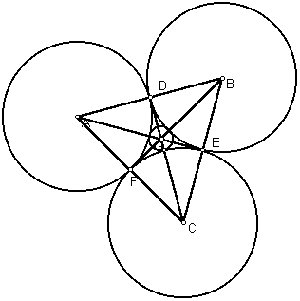We would be pleased if you find an error in the word problem, spelling mistakes, or inaccuracies and send it to us. Thank you!Tips to related online calculators

#### You need to know the following knowledge to solve this word math problem:

We encourage you to watch this tutorial video on this math problem:

## Next similar math problems:

• Common chordTwo circles with radius 17 cm and 20 cm are intersect at two points. Its common chord is long 27 cm. What is the distance of the centers of these circles?
• Eq triangle minus arcsIn an equilateral triangle with a 2cm side, the arcs of three circles are drawn from the centers at the vertices and radii 1cm. Calculate the content of the shaded part - a formation that makes up the difference between the triangle area and circular cuts
• Rectangle - parallelogram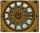It is given a rectangle that is circumscribed by a circle with a radius of 5 cm. The short side of the rectangle measures 6 cm. Calculate the perimeter of a parallelogram ABCD whose vertices are the midpoints of the sides of the rectangle.
• Equilateral triangle v3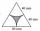Calculate the content of the colored gray part. Equilateral triangle has side length 8 cm. Arc centers are the vertices of a triangle.
• CircleWhat is the radius of the circle whose perimeter is 6 cm?
• CirclesIn the circle with a radius 7.5 cm are constructed two parallel chord whose lengths are 9 cm and 12 cm. Calculate the distance of these chords (if there are two possible solutions write both).
• Square and circlesThe square in the picture has a side length of a = 20 cm. Circular arcs have centers at the vertices of the square. Calculate the areas of the colored unit. Express area using side a.
• Circle annulusThere are 2 concentric circles in the figure. Chord of larger circle 10 cm long is tangent to the smaller circle. What are does annulus have?
• Triangle in circle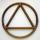Vertices of the triangle ABC lies on a circle with radius 3 so that it is divided into three parts in the ratio 4:4:4. Calculate the circumference of the triangle ABC.
• Four circles1) Calculate the circle radius if its area is 400 cm square 2) Calculate the radius of the circle whose circumference is 400 cm. 3) Calculate circle circumference if its area is 400 cm square 4) Calculate the circle's area if perimeter 400 cm.
• n-gon IIWhat is the side length of the regular 5-gon circumscribed circle of radius 11 cm?
• Chord MNChord MN of circle has distance from the center circle S 120 cm. Angle MSN is 64°. Determine the radius of the circle.
• Intersections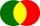How many intersections have circles with radius 16 mm and 15 mm, if the distance of their centers is 16 mm.
• Concentric circles and chordIn a circle with a diameter d = 10 cm, a chord with a length of 6 cm is constructed. What radius have the concentric circle while touch this chord?
• SectorThe perimeter of a circular sector with an angle 1.8 rad is 64 cm. Determine the radius of the circle from which the sector comes.
• Circle and hexagon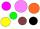Calculate the radius of a circle whose circumference is 8.4 cm longer than the circumference of the inscribed regular hexagon.
• Two circles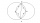Two circles with the same radius r = 1 are given. The center of the second circle lies on the circumference of the first. What is the area of a square inscribed in the intersection of given circles?